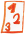Math worksheetsCustom math worksheets at your fingertips

# Details for problem "Insert relational operator"

Quickname: 7760

## Summary

Insert the correct relational symbol between two decimals.

## Example## Description

A series of pairs of decimal fractions is presented. The task is to determine whether these numbers are equal or not, if not, which one is greater, and insert the correct relational operator "less than", "greater than", or "equal" in the blank space between the numbers.

The way the pairs of numbers, a and b, are chosen can be selected from different algorithms:

- n and m are natural numbers from 1 to 9, a is n+m/10, b is n+m/100, i.e. m will occur in the tenth position of the first number a and in the hundredth position of the second number b. Example: 4.5 and 4.05.

- a is a natural number in the range from 11 to 99, b is a/10, e.g. 45 and 4.5.

- a and b are numbers with three decimals, a is by 0.001 larger than b.

- a is a number with one decimal place, b is b+0.001,..,0.09, e.g. 4.1 and 4.103.

- a and b have the same digits, but the order is different, e.g. 4.21 and 40.12.

- a and b are equal, but b is shown with a trailing zero, e.g. 4.2 and 4.20.

For each type of problem, the number of occurrences can be chosen. The problems of different types may occur grouped or shuffled, this is selectable.

Numbers may be positive or negative, this is configurable.

Download free worksheets for this math problem here. There is a second sheet with the solutions. Just click on the respective link.Worksheet 1Solution sheetWorksheet 2Solution sheetWorksheet 3Solution sheet

If you can not see the solution sheets for download, they may be filtered out by an ad blocker that you may have installed. If this is the case, please allow ads for this page and reload the page. The solution sheets will then reappear.

• Do the sample worksheets do not really fit?
• Do you need more math worksheets, with a different level of difficulty?
• Would you like to combine different problems on a worksheet and adjust them to your needs?
• As a teacher, you can put together your own worksheets using the automatically generated math problems provided.
With a free initial credit, you can start creating your own math worksheets in a few minutes.

It does not cost anything to try! Register here, to create custom worksheets now!

## Customization options for this problem

ParameterPossible values
# n,m natural,a=n+m/10,b=n+m/1000, 1, 2, 3, 4, 5, 6
# a=11-99, b=a/100, 1, 2, 3, 4, 5, 6
# a,b three decimals, a greater by 0.0010, 1, 2, 3, 4, 5, 6
# a one decimal, b three, tenths=00, 1, 2, 3, 4, 5, 6
# a und b jumbled digits0, 1, 2, 3, 4, 5, 6
# a,b same, b with trailing zero0, 1, 2, 3, 4, 5, 6
ShuffleYes, No
Positive/Negativepositive, across origin, positive/negative, negative